## Thursday, November 30, 2017

### Studying discrete-time dynamical systems (IV): generalization of dynamical systems able to produce spirals and clusters of points

Here is an animation of the families mentioned in my previous post: $$S_{D}=\{(x,y, f(z)): (x_{n+1},y_{n+1}) = (Im(f(x_{n} + y_n i)\cdot\sin{\frac{D}{f}}, Re(f(x_{n} + y_n i))\cdot\cos{\frac{D}{f}})\}$$ Where $f(z)=\frac{1}{(z+1)^t}, t$ from $1$ to $2$ by $0.01$ steps. Each frame is a complete family plot, calculated using $D \in [0,8 \cdot 10^3], n \in [0,8 \cdot 10^3]$ zoom into region $z=[+/-5]+[+/-5]i$. It took me some days to render it:

It is visually astonishing! Still I did not receive any feedback regarding my question at Mathematics Stack Exchange about the dynamical systems that make these beauties. As far as I know it is the first time they are shown.

## Monday, November 27, 2017

### Studying discrete-time dynamical systems (III): generalization of dynamical systems able to produce spirals and clusters of points

In a previous post I wondered if there were any other dynamical systems able to generate spirals and clusters of points as the found expression:
$$S_{D}=\{(x,y): (x_{n+1},y_{n+1}) = (Im(\psi(x_{n} + y_n i)\cdot\sin{\frac{D}{f}}, Re(\psi(x_{n} + y_n i))\cdot\cos{\frac{D}{f}})\}$$
To answer this, first I had a look to the image of the complete plot of the family of spirals:

We can see that there is a quasi-horizontal symmetry there. Both the "upper body" ($z=a+bi , b \ge 0$) and "lower body" ($z=a+bi , b \lt 0$) are quite symmetrical, but not exactly equal. The structure also has three vertical bodies / structures, a right side arc-like structure, a central body and a left-side body. These are the regions where the density of points is bigger.

The density of points make this structures to look like they are "glowing" (e.g. they remind a bioluminiscent jellyfish). The areas where there is more density of points seems to "glow" compared with other areas of lower density.

First question: who is responsible for making the spirals and who is responsible for deciding the location and distortion of the spirals?

To answer this, first I need to amend the expression:
$$S_{D}=\{(x,y): (x_{n+1},y_{n+1}) = (Im(\psi(x_{n} + y_n i)\cdot\sin{\frac{D}{f}}, Re(\psi(x_{n} + y_n i))\cdot\cos{\frac{D}{f}})\}$$ And make it more general, it is: $$S_{D}=\{(x,y, f(z)): (x_{n+1},y_{n+1}) = (Im(f(x_{n} + y_n i)\cdot\sin{\frac{D}{f}}, Re(f(x_{n} + y_n i))\cdot\cos{\frac{D}{f}})\}$$ So indeed: the family of dynamical systems is even bigger. Depending on the complex function that is used we will have a different structure. All of them have similar transformations but the results depend obviously on the selected function. So basically my original question was about the above more general family of dynamical systems when $f(z)=\psi(z)$ is specifically the digamma function.

Now, going back to the digamma expression:
$$S_{D}=\{(x,y): (x_{n+1},y_{n+1}) = (Im(\psi(x_{n} + y_n i)\cdot\sin{\frac{D}{f}}, Re(\psi(x_{n} + y_n i))\cdot\cos{\frac{D}{f}})\}$$ Let us split the expression into two versions:

Version 1: No sine or cosine are applied:
$$S_{D}=\{(x,y): (x_{n+1},y_{n+1}) = (Im(\psi(x_{n} + y_n i)), Re(\psi(x_{n} + y_n i)))\}$$and

Version 2: No function is applied, only the crossing of $Re$ and $Im$ (the meaning of "crossing" is as explained in the question):
$$S_{D}=\{(x,y): (x_{n+1},y_{n+1}) = (Im(x_{n} + y_n i)\cdot\sin{\frac{D}{f}}, Re(x_{n} + y_n i)\cdot\cos{\frac{D}{f}})\}$$ Version 1 will provide the following result:Version 2 will provide the following result (for the same $n$ iterations and $f$ and $D$ parameters):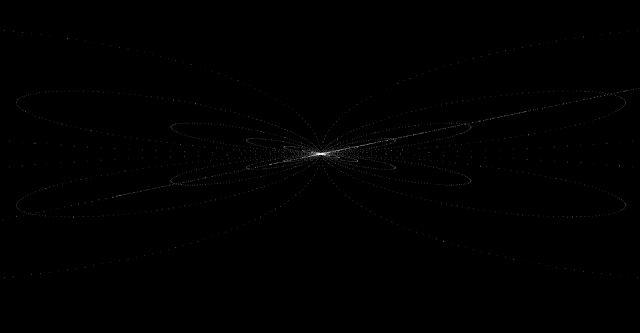So the conclusion is: the sine and cosine expressions provide the observed horizontal quasi-symmetry of the dynamical systems, and the digamma function was providing the spiral-like structures. The combination of both expressions provide a final structure in which the spirals of the digamma function are transformed, displaced and distorted by the sine and cosine transformations.
Second question: what do we obtain if we replace the digamma function by other functions?

My observations are that we obtain different "glowing" systems, but they share similar characteristics: horizontal quasi-symmetry, and three vertical structures (left, center, right), in other words, regions in which the density of points is bigger.

These are some of those beauties:

Scaled complementary error function, $f(z)=exp(z^2) \cdot erfc(z)$. (see Python scipy.special functions list, Steven G. Johnson, Faddeeva W function implementation). $D \in [0,10^4], n \in [0,10^4]$ zoom into region $z=[+/-5]+[+/-5]i$:$f(z) =$ Exponential integral $E_1$ of complex argument $z$. (see Python scipy.special functions list). $D \in [0,10^3], n \in [0,10^4]$ zoom into region $z=[+/-1]+[+/-4]i$: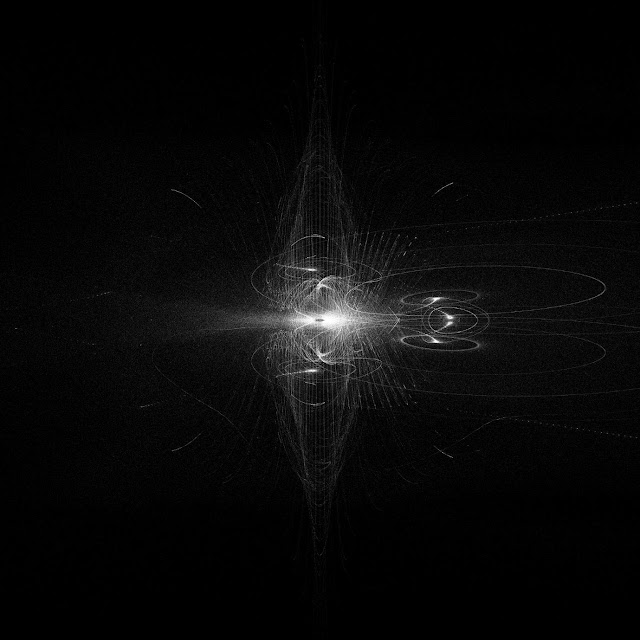$f(z)=$ Exponential integral $E_i$. (see Python scipy.special functions list). $D \in [0,10^3], n \in [0,10^4]$ zoom into region $z=[+/-5]+[+/-5]i$:Finally, my favorite families so far, $f(z)=\frac{1}{(z+1)^t}, t \in \Bbb R$. $D \in [0,8 \cdot 10^3], n \in [0,8 \cdot 10^3]$ zoom into region $z=[+/-5]+[+/-5]i$::

$f(z)=\frac{1}{(z+1)}$: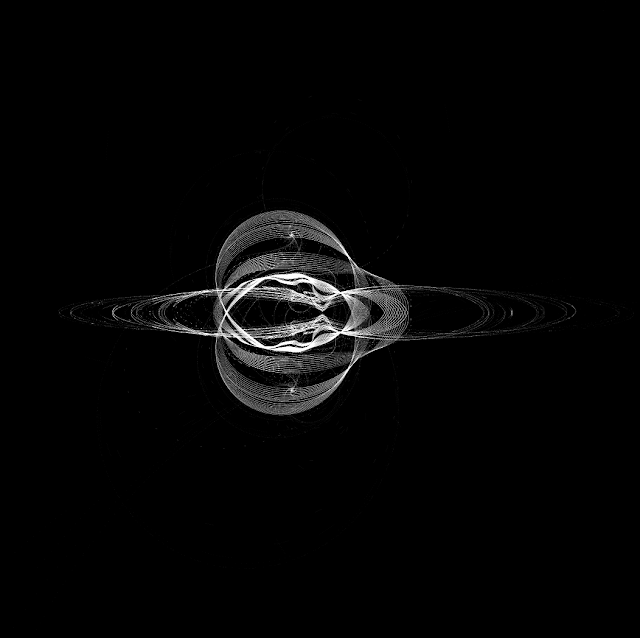$f(z)=\frac{1}{(z+1)^{1.1}}$:$f(z)=\frac{1}{(z+1)^{1.2}}$:$\cdots$

$f(z)=\frac{1}{(z+1)^{2}}$: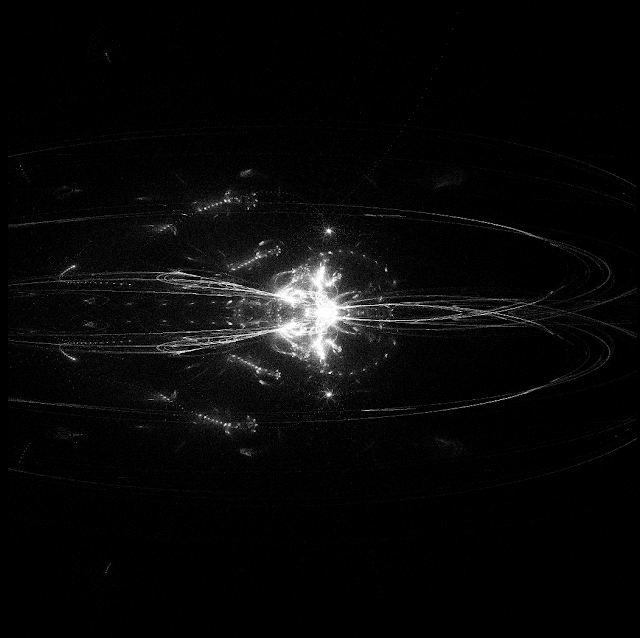$\cdots$

$f(z)=\frac{1}{(z+1)^{3}}$:I just can say that these structures are visually beautiful. I will keep my question regarding these families of dynamical systems open at MSE in hope that somebody will be able to provide more insights about why this transformation / mapping works in this way.

## Wednesday, November 15, 2017

### Trying to understand the maths behind the movements of a two-legged air dancer (aka skydancer, tube man)

I am trying to understand the maths behind the movements of a two-legged air dancer, aka skydancer aka tube man. Well, I mean these cheery friends here:(This is a mirror of my question on this topic at MSE) The following discrete time algorithm is my devised solution, but I am sure that other much better approaches are possible. Indeed it is not very realistic and I am not very happy. I would like to learn other solutions more closer to reality and related with dynamical systems, complex dynamics or fluid dynamics:

1. Take for instance a unit square, same width and height. Then generate two partially (explanation of the "partially" wording is done below) random walks with these rules:

2. The first partially random walk $R1$ starts at $(0,0)$ and finishes at $(1,1)$

3. The second random walk $R2$ starts at $(0,1)$ and finishes at $(1,0)$

4. We will generate $n$ random points sequentially for $R1$ as follows. The first point is $(x_0,y_0)=(0,0)$, then we will take a random point from the intervals $([0,1],[0,1])$, it will be $(x_1,y_1)$. Then $(x_2,y_2)$ will be a random point from the intervals $([x_2,1],[y_2,1])$, then next point $([x_3,1],[y_3,1])$, etc. So we are approaching $(1,1)$ on each iteration. Usually $n=20$ iterations is good enough to be visually very close to $(1,1)$ so the simulation is good enough. The reason of the "partially" wording is that on every iteration we reduce the intervals, so it is not always a random point of the square unit, so it is not totally random.

5. In the same fashion we will generate points for $R2$ but the intervals will approach on each iteration to $(1,0)$.

6. Now we will create segments joining the sequential points of $R1$ and same for $R2$.

7. As both paths are crossing completely each diagonal of the square unit, there is an intersection point between them. Mark with a disk the intersection of both random paths and display the unit square including the segments and the intersection.

8. Execution: the algorithm can be looped for an infinite time of $t$ units in a loop, and each iteration $t$ will generate the aspect of the air dancer for that unit of time. So in my case the algorithm makes a kind of discrete system.

And this is how looks my air dancer simulation: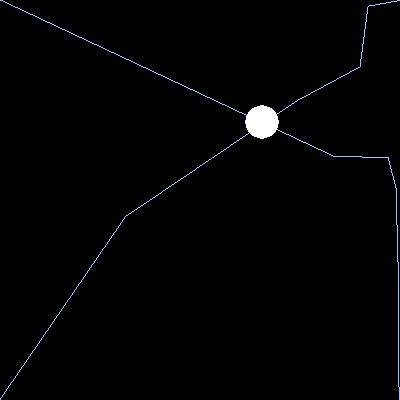And another one with two dancers:Some facts and trivia:

1. Due to the way that the segments are generated, the lengths of them are not totally random, see the square line picking problem.

2. The above solution might be defined as a kind of discrete map where each unit of time $t$ is independent of the status of the previous unit of time (but this can be modified to make movements depend on the previous unit of time at some extent so it would be a standard discrete-time map).

3. The algorithm works for any square, not only the unit square, it is just an example. They can be any desired width and height.

4. The legs of the air dancers are usually fixed to the ground, but not the arms, and that is a part of my simulation that is not very realistic (apart from others) although I think that it would be more or less easy to enhance this point specifically.

I would like to simulate this kind of objects with a more realistic approach, but initially I did not find a reference on Internet. My guessing is that it must be very related to dynamical systems and more specifically to fluid dynamics, so it might be possible to express the movement by a discrete (map) or continuous (based on derivatives) dynamical-system.

Below is the Python code for my simulation (also available at my account at repl.it). It will work in, for instance, a personal computer using Python Anaconda if the pygame library is downloaded as usual. Feel free to use and modify it and enjoy!

#!/usr/bin/env python
import pygame
import random
from collections import defaultdict
from time import sleep

# This script aims to simulate a tube man aka air dancer aka skydancer
# https://en.wikipedia.org/wiki/Tube_man

# The code will generate a TOTWIDTHxTOTHEIGHT pixels screen
# where HOBJECTSxVOBJECTS skydancers are generated
# each one with a similar WIDTHxHEIGHT
# the body of each skydancer is a disk of radius = RBIG pixels
# the simulation runs until ESC is pressed and each
# simulation time unit is shown during DELAYSEC seconds
# then the screen is wiped and the next time unit image is calculated

# Initialization constraints
HOBJECTS=2 #number of air dancers per line
VOBJECTS=1 #number of rows
WIDTH, HEIGHT = 200, 200
TOTWIDTH, TOTHEIGHT = WIDTH*HOBJECTS, HEIGHT*VOBJECTS
RBIG = 17#10#17
RSMALL = 8#8
DELAYSEC=0.3

def air_dancer_simulator():
pygame.init()
win=pygame.display.set_mode((TOTWIDTH, TOTHEIGHT))

keysPressed = defaultdict(bool)

def ScanKeyboard():
while True:
# Update the keysPressed state:
evt = pygame.event.poll()
if evt.type == pygame.NOEVENT:
break
elif evt.type in [pygame.KEYDOWN, pygame.KEYUP]:
keysPressed[evt.key] = evt.type == pygame.KEYDOWN

# detects the intersection point (x,y) of two lines, if exists.
def line_intersection(line1, line2):
xdiff = (line1 - line1, line2 - line2)
ydiff = (line1 - line1, line2 - line2)

def det(a, b):
return a * b - a * b

div = det(xdiff, ydiff)
if div == 0:
raise Exception('lines do not intersect')

d = (det(*line1), det(*line2))
x = det(d, xdiff) / div
y = det(d, ydiff) / div
return x, y

bClearScreen = True
pygame.display.set_caption('Skydancer aka tube man aka air dancer simulator')
loop=0

#Seed points of the air dancer sequential segments to be generated are (0,0) and (0,1)
x_currpos_incdiag = 0
y_currpos_incdiag = 0
x_currpos_decdiag = 0
y_currpos_decdiag = HEIGHT-1

test_points_inc=[[x_currpos_incdiag,y_currpos_incdiag]]
test_points_dec=[[x_currpos_decdiag,y_currpos_decdiag]]

frames=0
# the simulation will run infinite discrete units of time (ESC to finish the simulation)
while True:
# draw biash
for biash in range(0,HOBJECTS):
for biasv in range(0,VOBJECTS):
loop=1
while True:
# each dancer is finished in 20 iteracions
if loop%20==0:

found=False
for i in range(0,len(test_points_inc)-2):
for j in range(0,len(test_points_dec)-2):
try:
x,y=line_intersection([test_points_inc[i],test_points_inc[i+1]],[test_points_dec[j],test_points_dec[j+1]])
if x>=0 and x<=WIDTH and y>=0 and y<=HEIGHT:
if (test_points_inc[i]<=x) and (x<=test_points_inc[i+1]):
if (test_points_inc[i]<=y) and (y<=test_points_inc[i+1]):
if (test_points_dec[j]<=x) and (x<=test_points_dec[j+1]):
if (test_points_dec[j]>=y) and (y>=test_points_dec[j+1]):
# Detected interection of two segments, will be the body of the air dancer
pygame.draw.circle(win, (255,255,255),(int(x)+(biash*WIDTH),int(y)+(biasv*HEIGHT)),RBIG)
found=True
break
except:
pass

if found==True:
break

#pygame.display.flip()
#sleep(5)

#initial positions
x_currpos_incdiag = 0
y_currpos_incdiag = 0
x_currpos_decdiag = 0
y_currpos_decdiag = HEIGHT-1
test_points_inc=[[x_currpos_incdiag,y_currpos_incdiag]]
test_points_dec=[[x_currpos_decdiag,y_currpos_decdiag]]
break

x_newpos_incdiag = random.randint(x_currpos_incdiag,WIDTH-1)
y_newpos_incdiag = random.randint(y_currpos_incdiag,HEIGHT-1)
x_newpos_decdiag = random.randint(x_currpos_decdiag,WIDTH-1)
y_newpos_decdiag = random.randint(0,y_currpos_decdiag)

test_points_inc.append([x_newpos_incdiag,y_newpos_incdiag])
test_points_dec.append([x_newpos_decdiag,y_newpos_decdiag])

pygame.draw.line(win, (150,200,255),(x_currpos_incdiag+(biash*WIDTH),y_currpos_incdiag+(biasv*HEIGHT)),(x_newpos_incdiag+(biash*WIDTH),y_newpos_incdiag+(biasv*HEIGHT)))
pygame.draw.line(win, (150,200,255),(x_currpos_decdiag+(biash*WIDTH),y_currpos_decdiag+(biasv*HEIGHT)),(x_newpos_decdiag+(biash*WIDTH),y_newpos_decdiag+(biasv*HEIGHT)))

# Add horizontal and vertical lines too
#pygame.draw.line(win, (255,255,255),(x_currpos_incdiag+(biash*WIDTH),y_currpos_incdiag+(biasv*HEIGHT)),(x_currpos_incdiag+(biash*WIDTH),0+(biasv*HEIGHT)))
#pygame.draw.line(win, (255,255,255),(x_currpos_incdiag+(biash*WIDTH),y_currpos_incdiag+(biasv*HEIGHT)),(x_currpos_incdiag+(biash*WIDTH),HEIGHT+(biasv*HEIGHT)))
#pygame.draw.line(win, (255,255,255),(x_currpos_decdiag+(biash*WIDTH),y_currpos_decdiag+(biasv*HEIGHT)),(0+(biash*WIDTH),y_currpos_decdiag+(biasv*HEIGHT)))
#pygame.draw.line(win, (255,255,255),(x_currpos_decdiag+(biash*WIDTH),y_currpos_decdiag+(biasv*HEIGHT)),(WIDTH+(biash*WIDTH),y_currpos_decdiag+(biasv*HEIGHT)))

# Add circles on each beginning and end of segment
#pygame.draw.circle(win, (150,200,255),(x_currpos_incdiag+(biash*WIDTH),y_currpos_incdiag+(biasv*HEIGHT)),RSMALL)
#pygame.draw.circle(win, (150,200,255),(x_currpos_decdiag+(biash*WIDTH),y_currpos_decdiag+(biasv*HEIGHT)),RSMALL)

x_currpos_incdiag = x_newpos_incdiag
y_currpos_incdiag = y_newpos_incdiag
x_currpos_decdiag = x_newpos_decdiag
y_currpos_decdiag = y_newpos_decdiag

win.lock()
win.unlock()
ScanKeyboard()

if keysPressed[pygame.K_ESCAPE]:
pygame.image.save(win, "intersections.png")
break

loop = loop+1

if keysPressed[pygame.K_ESCAPE]:
pygame.image.save(win, "intersections.png")
break

if keysPressed[pygame.K_ESCAPE]:
pygame.image.save(win, "intersections.png")
break

if keysPressed[pygame.K_ESCAPE]:
pygame.image.save(win, "intersections.png")
break

if keysPressed[pygame.K_ESCAPE]:
pygame.image.save(win, "intersections.png")
break

pygame.display.flip()
sleep(DELAYSEC)

# Uncomment to save the frames, then you can create an animated gif or video with
# your favorite program (e.g. VirtualDub)
#pygame.image.save(win, "airdancer_" + str(frames) + ".png")

frames=frames+1

if bClearScreen:
win.fill((0, 0, 0))

air_dancer_simulator()

## Monday, November 6, 2017

### Studying discrete-time dynamical systems (II): a dynamical system able to produce spirals and clusters of points

I have found by trial and error an interesting family of dynamical systems giving some nice strange attractors. They are chaotic complex systems based on the digamma function. It is defined by a complex discrete map as follows:
(This is a mirror of my question at MSE)

$$S_{D}=\{(x,y): (x_{n+1},y_{n+1}) = (Im(\psi(x_{n} + y_n i)\cdot\sin{\frac{D}{f}}, Re(\psi(x_{n} + y_n i))\cdot\cos{\frac{D}{f}})\}$$

Where:

1. The seed is $(x_0,y_0)=(\pi/4,\pi/4)$

2. $\psi(x_{n} + y_n i)$ is the digamma function applied to a complex number $z_n=x_n+y_n i$

3. $f$ is a fraction constraint initially for this test fixed to $f = 1$.

4. $D$ is a constraint called "Depth", $D \in \Bbb N$.

5. The process is just calculating on each iteration the digamma function and then generating a complex number $(z_{n+1}=x_{n+1},y_{n+1})$, where the real part of the complex number at the iteration $n+1$ comes from the imaginary part of the complex number associated with the previous iteration $n$, and likewise the imaginary part of the complex number of the iteration $n+1$ comes from the real part of the complex number generated at the iteration $n$. In other words, it is "crossing" $Im$ and $Re$ on each iteration.

Some details of the trial and error process: Any other combination of sine, cosine or not "crossing" $Im$ and $Re$ on each iteration will not provide the patterns I will show below. Modifying the seed will provide the same results but located in other points of the plane or distorted. Modifying the fraction $f$ just generates other similar attractors, so $f$ was fixed to focus the tests.

Basically: for every fixed $D$ (Depth) and for a fixed $f=1$ value, if we run the system up to as much as we want big $n$ we arrive to a sink of a strange attractor, so from that point the set of points will not grow, and the graph of the set of points $S_n$ shows an attractor.

Running the system for $D \in [0,250]$ and up to $n = 4 \cdot 10^4$ (it can be a higher value but for the tests is a good enough number) we obtain a family of attractors that can be divided into three different types or shapes: **clusters**, **spirals** and what I call "**anomalies**".

1. Clusters are just clusters of points, a rounded shape, but the points can not escape from that space. For instance, $S_5$:

2. Spirals have the shape of different types of spirals: two arms, three arms, four, etc. For instance, $S_{45}$:

3. Anomalies are very specific shaped attractors. The limits are very well defined. For instance, $S_{22}$ and $S_{75}$:

I wanted to see all them together, they look like this, it is zoomed a little bit:There is too much density of points when we show the big clusters together with the spirals and the anomalies, so I decided to split them into three groups and I focused on the family of spirals, and this is the graph of the spiral-shaped attractors in $Depth \in [0,250]$ (click to zoom the image in a full screen view):I wanted to make a simulation exercise with this. As it is said that complex dynamical systems are closer to reality and the attractors obtained are spirals and clusters I tried to see if it is possible to simulate some galaxies and star clusters with this information. So I added a third dimension using the $Depth$ value.
$$S_{Depth}=\{(x,y,d): (x_{n+1},y_{n+1},d_{n+1}) = (Im(\psi(x_{n} + y_n i)\cdot\sin{\frac{D}{f}}, Re(\psi(x_{n} + y_n i))\cdot\cos{\frac{D}{f}}, D+(\sin{n}))\}$$

The $d$ value locates each attractor $S_D$ in a different depth, and the sine is just a trick to add some "random" volume to each cloud of points of each attractor. I used a LiDAR free viewer to visualize the $(x,y,d)$ points in the three-dimensional space (in my case PointCloudViz Free Edition), and it looked quite promising. I decided to invert and colorize the images with GIMP2, and these are some results. They are exactly the same points as shown in the previous plots, but colorized and viewed from inside the cloud of points in a three-dimensional environment (click to zoom the images in a full screen view):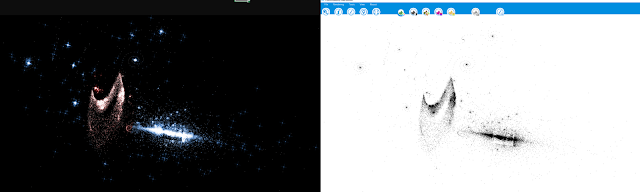It seems that these points can "simulate" galaxies at some rudimentary extent. It would be interesting to run a n-body simulation with this family of attractors.

I am not sure if it is correct to talk about strange attractors in the case of the cluster and spiral shapes. The iterations really arrive to a $n$ value such that the point $(x_n,y_n)$ is a sink and the set of points does not grow from that moment, but I am not sure if it is correct to talk about strange attractor, just attractor or just dynamical system attached to a complex map. I am more sure about for instance the case $D=22$ shown above because the shape of the attractor is very clear.

I wonder if there are any other dynamical systems able to generate this kind of shapes.

update 2017/11/10: for the sake of completeness, below there is a two dimensional $n$-body gravity simulation using the first $600$ points generated by the $S_5$ set. We consider the points as a protoplanetary disk, and the closest point to the average center of the points will be given the biggest density and thus it will turn into a central sun. I have provided random densities, radius, mass and momentum to the rest of points, so they simulate distinct types of planets by accretion. The less dense planets are whitish-bluish, the average density planets are greenish (Earth-like) and the heaviest planets are reddish. Depending on the volume, the colors are lightest on each variety, the bigger the lighter. When two planets collide, the bigger mass takes the average density of both planets and all the mass and momentum. When the planet collides with the central sun, the sun takes all the mass and the average density but it remains fixed as the center of the system. This simulation (is a looped animation) has $12500$ units of time. The Python code is a very nice work by Thanassis Tsiodras, with some customizations of my own to add random densities and colors for a little bit more realistic visualization, so credits to the author (site here):
It seems that the clouds of points generated by the dynamical system are valid to simulate some basic behavior of protoplanetary disks. We can see that the initial accretion cloud starts to be cleaned out: some protoplanets collide and generate a bigger planet with a new averaged density and radius. Others are expelled from the protoplanetary disk due to the distinct gravity interactions. Others collide with the sun, making it bigger, and finally after some units of time it gets stable with some few planets of different density and more or less stable trajectories. Be aware that the animation is gradually zooming out to see the more external trajectories (it seems that the planets and the sun are shrinking because there is no background reference).

Update 2017/11/20: I have uploaded the code to my repl.it account (link here). It cannot be run online because it requires saving the images of the different dynamical systems. It will work in, for instance, a personal computer using Python Anaconda. Feel free to use and modify it and enjoy! Just in case, here is a backup copy too:

# Why is this family of dynamical systems able to produce spirals and clusters of points?
# code attached to the question at Mathematics Stack Exchange: https://math.stackexchange.com/q/2506900/189215
# keys: recurrence-relations, dynamical-systems, visualization, experimental-mathematics, chaotic-systems

def sa():
import matplotlib.pyplot as plt
from math import pi
from cmath import cos , sin
from scipy.special import digamma

# Each system is iterated up to [testlimit] times
# It can be a higher value but for the tests is a good enough number to arrive to the sink points
testlimit = 40000 #1000000

# Complete list of points of each system, the first point of the list is the (x,y,z) seed
# each dynamical system will have up to [testlimit] points. If we arrive to a sink point before the [testlimit]
# iteration there is not need to continue, so the quantity of elements will be smaller.
st=[]

# Splitted lists of x,y and z coordinates of the points obtained on each iteration of the system stored at the [st] list
lox = []
loy = []
loz = []

# Depth D parameter range
rangestart = 0
rangelimit = 250

# f parameter definition
fraction=1

# Using this very code, and reviewing the intependent graphs of the result of each
# dynamical system, it is possible to split them into three different type of systems
# anomalies, big clusters and spirals, see explanation at https://math.stackexchange.com/q/2506900/189215
# you do not know beforehand (without printing) which Depth D value gives an anomalie, big cluster or spiral type
# system, so the code below was added after printing individually each system and then splitting them into the
# three possible types. So it was made by trial-error.
anomalies_list = [22,25,44,47,66,88,97,110,113,132,135,151,173,176,179,195,198,204,220]
bigclusters_list = [5,8,11,16,27,30,33,38,49,52,55,60,71,74,77,79,82,93,96,99,104,115,118,121,126,137,140,143,147,148,159,162,165,169,170,180,181,184,187,192,202,203,206,209]

# We will generate a dynamical system for each Depth D parameter from [rangestart] to [rangelimit] included.
for depth in range (rangestart,rangelimit+1):

# Uncomment to avoid plotting the anomalies
#if depth in anomalies_list:
#    continue

# Uncomment to avoid plotting the big clusters
#if depth in bigclusters_list:
#    continue

st=[]

# Seed of the dynamical system
st.append([pi/4,pi/4,depth])

# We will generate up to [testlimit] points of the current dynamical systes for a fixed D Depth.
# if we arrive to a sink point we do not need to continue the loop up to [testlimit] because the
# point will be repited again (so we do not need to plot more than once the sink point)
for n in range (1,testlimit):

# We read the former the previous iteration point (oldx,oldy,oldz)
oldx=st[len(st)-1]
oldy=st[len(st)-1]
oldz=st[len(st)-1]

# Then we calculate the digamma function of the previous iteration complex point [oldx+(oldy*(1j)]
c=digamma(oldx+(oldy*(1j)))

# This is the new point of the dynamical system for the current iteration
newx=(c.imag)*(sin(depth/fraction))
newy=(c.real)*(cos(depth/fraction))
# The z coordinate is just a trick to give a random depth in space to the points
# so we can simulate at some rudimentary extent a "physical" galaxy with the (x,y,z) points later
newz=depth+(sin(n)).real

# We add it to the list [st] of points (x,y,z) of the current dynamical system
st.append([newx.real,newy.real,newz])

# Once we have generated the points of the current D, f dynamical system, we have a
# set of points S_D (where D is the Depth number). E.g S_1, S_2, etc.
# see examples at https://math.stackexchange.com/q/2506900/189215
# this loop below will remove repeated points before plotting them
# to avoid memory overflow
for idx in range(0,len(st)-1):
if len(lox)==0:
lox.append(st[idx])
loy.append(st[idx])
loz.append(st[idx])
elif not(str(lox[len(lox)-1])==str(st[idx]) and (str(loy[len(loy)-1])==str(st[idx]))):
# if we arrive to a sink we cannot go out from it, so we do not need to add again the same point
lox.append(st[idx])
loy.append(st[idx])
loz.append(st[idx])

# we set black color to the background and white color to the points
ax = plt.gca()
ax.set_axis_bgcolor((0, 0, 0))
figure = plt.gcf()

# If we want to see accuracy and details, we need to use a big enough resolution like the one below
# Uncomment if you want to use the ZOOM-IN code
#figure.set_size_inches(320, 32)
#figure.set_size_inches(20, 32)

##ZOOM-IN print code BEGIN

#if depth==rangelimit:
#    # If we have generated all the family of dynamical systems, then we will print them all together
#    plt.plot(lox,loy,",w")
#    # Uncomment if you just want to diplay them
#    #plt.show()
#
#    # This loop will generate some png files making a zoom-in to the plot
#    # of the family of dynamical systems. You can joint them and create
#    # an animation (div, anigif) with for instance the free application [VirtualDub]
#    # the values and limits of the zoom were calculated by trial and error
#    for zoom in range (105,140):
#        dim=37/(zoom/100)
#        plt.axis([-dim,dim,-dim*4/37,dim*4/37])
#        plt.savefig("dynamical_system_digamma_family_zoom_in_"+str(zoom)+".png")
##ZOOM-IN print code END

# We will show the current Depth D generated dynamical system
plt.plot(lox,loy,",w")
#plt.show()
#We will save each dynamical system in a png file
plt.savefig("dynamical_system_digamma_family_depth_"+str(depth)+".png")

# then we clean the buffers and lists
# If you want to use the ZOOM-IN code bove please COMMENT the code below (BEGIN-END)
# BEGIN Clean buffers
plt.clf()
lox = []
loy = []
# END Clean buffers

sa()